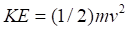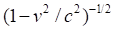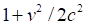Chapter 12, Problem 1C

Chapter
Section
Textbook Problem

Show that in the limit of low speeds the expression for the relativistic kinetic energy (see Important Equations) reduces to the familiar one from classical mechanics,[Hint: For speeds v much smaller than c, the quantityis approximately equal toYou should check this for yourself using typical speeds given inthe exercises in Chapter 3.]

To determine

To show:

The expression for relativistic kinetic energy is similar to classical kinetic energy in the limit of low speeds.

Explanation

Given data:

Rest mass of an object = mo

Speed of the object = v.

Calculation:

Relativistic kinetic energy is written as

K=moc2 1 v 2 c 2 moc2K=moc2(1 1 v 2 c 2 1)K=moc2( ( 1 v 2 c 2 ) 1 2 1)by binomial approximation,  (1+

Still sussing out bartleby?

Check out a sample textbook solution.

See a sample solution

The Solution to Your Study Problems

Bartleby provides explanations to thousands of textbook problems written by our experts, many with advanced degrees!

Get Started